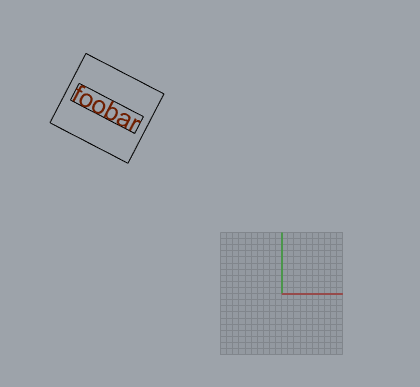# Bug TextEntity.GetBoundingbox()

Hi,

It seems there is a bug in getting the boundingbox of a TextEntity:The bigger box is when the geometry is left in place and the boundingbox is calculated relative to it’s own plane.

The smaller box is when the boundingbox is calculated from the geometry that is first orientated to the WorldXY

script to test on a rotated textobject:

``````import scriptcontext as sc
import Rhino

id = rs.GetObject('select text',preselect=True)
geom = rs.coercegeometry(id)

#transforms worldXY - geometry plane
from_geom = Rhino.Geometry.Transform.PlaneToPlane(geom.Plane, Rhino.Geometry.Plane.WorldXY)
to_geom = Rhino.Geometry.Transform.PlaneToPlane(Rhino.Geometry.Plane.WorldXY , geom.Plane)

#First get bbox from current orientation
bbox = geom.GetBoundingBox(geom.Plane)
rect = Rhino.Geometry.Rectangle3d(Rhino.Geometry.Plane.WorldXY,bbox.Min , bbox.Max)
rect.Transform(to_geom)

#Second get bbox from orienation on XYplane
geom.Transform(from_geom)
bbox = geom.GetBoundingBox(geom.Plane)
rect = Rhino.Geometry.Rectangle3d(Rhino.Geometry.Plane.WorldXY,bbox.Min , bbox.Max)
rect.Transform(to_geom)
``````

Am I being dense and missing something or is this indeed a bug?

@dale could you maybe shed some light on this?
Thanks
-Willem

1 Like

Hi @Willem,

Yeah, this is known.

https://mcneel.myjetbrains.com/youtrack/issue/RH-46608

The only “work around” I know of is to expode the text into curve and calculate the bounding box of the curves.

– Dale

1 Like

Thank you for the confirmation Dale.

-Willem